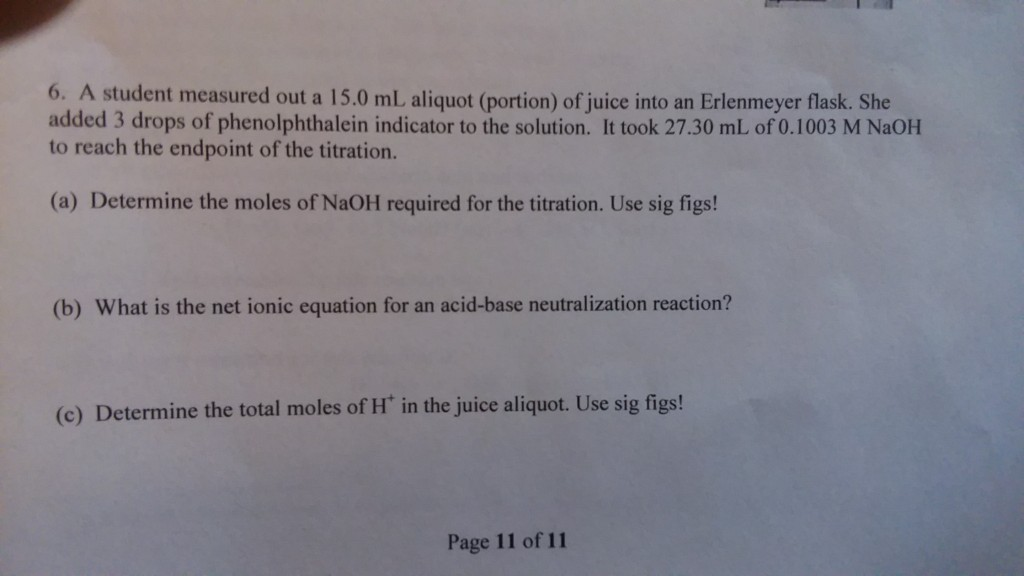# Question & Answer: A student measured out a 15.0 mL aliquot (portion) of juice into an Erlenmeyer flask…..A student measured out a 15.0 mL aliquot (portion) of juice into an Erlenmeyer flask. She added 3 drops of phenolphthalein indicator to the solution. It took 27.30 mL of 0.1003 M NaOH to reach the endpoint of the titration. (a) Determine the moles of NaOH required for the titration. Use sig figs! (b) What is the net ionic equation for an acid-base neutralization reaction? (c) Determine the total moles of H^+ in the juice aliquot. Use sig figs!

Given

Don't use plagiarized sources. Get Your Custom Essay on
Question & Answer: A student measured out a 15.0 mL aliquot (portion) of juice into an Erlenmeyer flask…..
GET AN ESSAY WRITTEN FOR YOU FROM AS LOW AS \$13/PAGE

a) 27.30 ml NaOH i.e. = 27.30 /1000 = 0.0273 L & 0.1003 M NaOH

Num of mole of NaOH = Molarity x Volume in liter = 0.1003 x 0.0273 = 0.002738 mole of NaOH

Num of mole of NaOH= 0.002738 moles

b) this is simple acid base neutralisation reaction

HX + NaOH  NaX + H2O

c) In this reaction the number of mole of NaOH require for neutralise Number mole od H+

so Mole of NaOH = Mole of H+

Mole of H+ = 0.002738 moles.